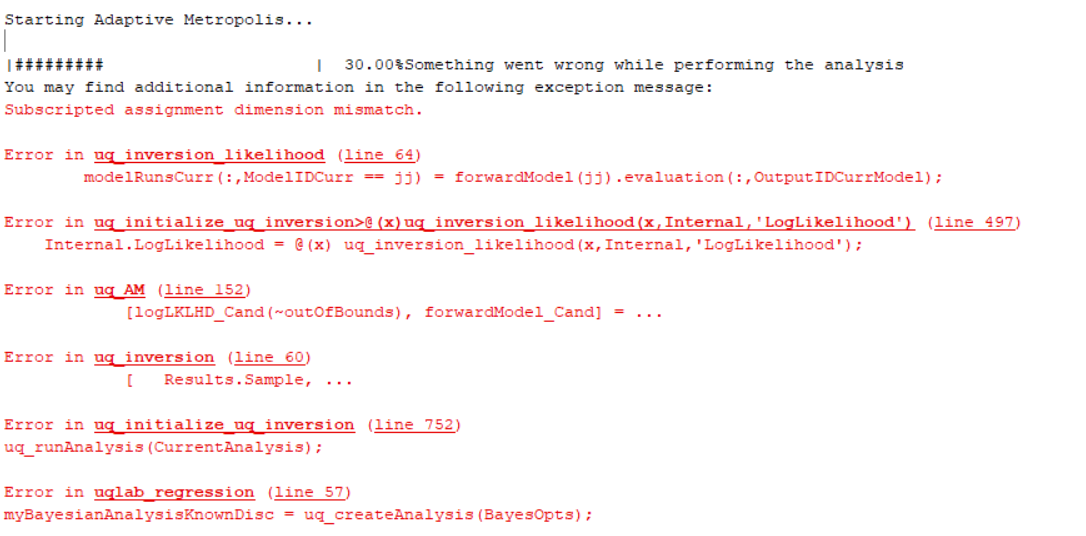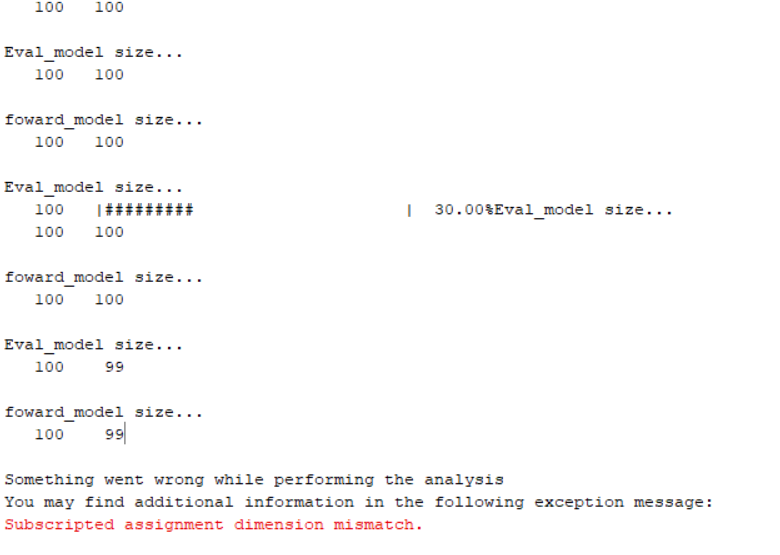# Shape Error during inference

Hi! I’m trying to implement Bayesian linear regression. Since the example given was kinda strange in terms of implementation I played around with the function handle in order to perform the inversion.
What I came up with is the following:

nsamples = 10;
N_cycles = log(linspace(1000, 1e7));
size_ =  size(N_cycles);
a = -10;
b = 1000;

big_Array = ones(size_(2),2);
big_Array(:,1) = N_cycles;

modelOpts.mHandle = @(x) big_Array * transpose(x);
modelOpts.isVectorized=true;
foward_model = uq_createModel(modelOpts);


I’m trying to set up linear regression for the Baskin relationship for the fatigue analysis of a mechanical component.
The idea behind it is that I observe \sigma = [\sigma_1,...,\sigma_n]^t observations linearly related to N the amount of cycles to failure. Then I try to get the distributions for a,b the parameters of the model.
When trying to perform the inference, the sampling algorithm starts and after a certain amount of samples throws the following error:Which is strange since it’s a shape error when the sampler already started. I believe the cause of this error to be in the settings I gave the sampler but I’m at a lost of what could it be.

Update:
The script raising the error has the following assignments (I’ve added the disp statements for debugging):

for ii = 1:Internal.nForwardModels
%retreive relevant model params
modelParamsCurr = modelParams(:,Internal.ForwardModel_WithoutConst(ii).PMap);

%forwardModel(ii).evaluation =
%uq_evalModel(Internal.ForwardModel_WithoutConst(ii).Model,modelParamsCurr,'hpc');

eval_model = uq_evalModel(Internal.ForwardModel_WithoutConst(ii).Model,modelParamsCurr);
disp('Eval_model size...')
disp(size(eval_model))

forwardModel(ii).evaluation = eval_model;
disp('foward_model size...')
disp(size(forwardModel(ii).evaluation))

end


As I run the code the following happens:Suddenly shape changes and then the shape error gets raised. This is quite mind-boggling but I believe it should make sense for someone more in touch with the algorithm.

Can you share a minimal working example? This way I’ll be able to easily reproduce your problem.